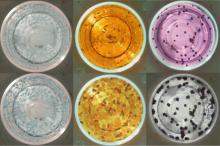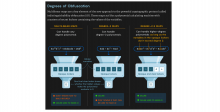# Faster Linear Equation Solving

Image: Quanta Magazine, Myriam Wares

Richard Peng and Santosh Vampala of Georgia Institute of Technology have developed a new method of solving linear equations that is faster than matrix multiplication. Quanta magazine published an interesting article describing the new method, how it differs from standard matrix multiplication using coordinated random guessing, and how it can solve any sparse linear equation in n2.332 steps compared to the n2.372 of matrix multiplication.

## Similar Items